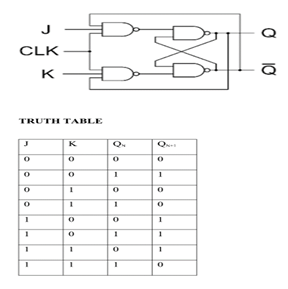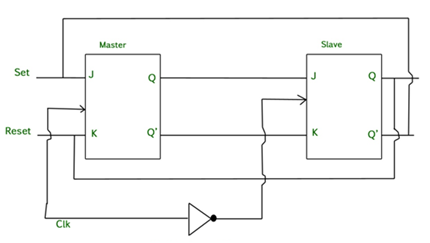Goseeko blog# What is Race around Condition?

Race around condition occurs in JK Flip Flop  when both its inputs J=K=1 and also the clock =1 for a long period.

J-K Flip Flop

The truth table is shown below with its circuit diagram.## Race Around Condition in JK Flip-flop

• For J-K flip-flop, if J=K=1, and if clk=1 for a long period of time, then output Q will toggle as long as CLK remains high which makes the output unstable or uncertain.
• This is called a race around condition in J-K flip-flop.
• We can overcome this problem by making the clock =1 for very less duration.
• The circuit used to overcome race around conditions is called the Master Slave JK flip flop.

Master Slave JK flip flop

• Here two JK flip flops are connected in series.
•  The first JK flip flop is called the “master” and the other is a “slave”.
•  The output from the master is connected to the two inputs of the slave whose output is fed back to inputs of the master.
•  The circuit also has an inverter other than the two flip flops.
• The Clock Pulse and inverter are connected because of which the flip flops get an inverted clock pulse.
•  In other words, if CP=0 for a master flip-flop, then CP=1 for a slave flip-flop and vice versa.## Working of a Master Slave flip flop

• When the clock pulse goes high, the slave is isolated; J and K inputs can affect the state of the system. The slave flip-flop is isolated when the CP goes low.
• When the CP goes back to 0, information is transmitted from the master flip-flop to the slave flip-flop and output is obtained.
• As the master flip flop is positive triggered it responds first and the slave later (it is negative edge triggered).
• The master goes to the K input of the slave when both inputs J=0 and K=1, and also Q’ = 1. In this case the slave copies the master as the clock forces the slave to reset.
• The master goes to the J input of the slave when both J=1 and K=0, Q = 1. The clock is set due to the negative transition of the clock.
• There is a state of toggle when both J=1 and K=1. On the negative transition of clock slave toggles and  the master toggles on the positive transition of the clock.
• Both the flip flops are disabled when both J=0 and K=0 and Q is unchanged.

Interested in learning about similar topics? Here are a few hand-picked blogs for you!